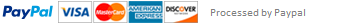### Python Programming Assignment

1. For these problems, a Boolean expression: means an expression like “2 < x <= 4” or “5<x and y>2”

a. If r and s are both numbers: write the Boolean expression that will make the statement that (r – s) is greater than -4 and less than 5.

b. if r = 8 and s = 2, what value would Python assign to the expression you wrote in part a. ?

c. if x=5,y=2, and z=False, what value will Python return if you type in this sentence: z == True and x < 4 or y >1

d. Write one Boolean expression that says the numbers n and r are both between 1 and 6 inclusive.

e. Write one Boolean expression that says the numbers n and r are either or both less than or equal to 4

2. Simplify using De Morgan’s laws: Note: the idea is to get rid of the not in each expression by using De Morgan’s law and then use a Boolean expression to express the a and b equalities and inequalities.

a. not (a >= 3 and b <= -2)

b. not(b<4 or a>2)

3. For the following problem submit a photo of the flowchart and shots of your Python screens showing the program code and the output. Your task is to input from the teacher the test score of each student and to output the highest score. You need to check each score to make sure it is valid (at least 0 and at most 100). If it is not valid, keep prompting for a valid score until you get one. After a valid score is input, process it, and then ask the teacher if she has another score to enter.

When you run the program, test the logic when the score entered is invalid, when it is a valid score, and when the teacher says she has no more scores to enter.

4. For the following problem submit a photo of the flowchart and shots of your Python screens showing the program code and the output. There are 6 students in the group. Ask each student to enter the number of minutes it took them to complete the homework. Only accept a non-negative number. If the number entered is negative, keep prompting until a non-negative number is entered. Find the average number of minutes to complete homework. Print a message that includes the average number of minutes.

Check the logic of your code by seeing what happens when you enter an invalid number of minutes and when you enter a valid number of minutes.

This assignment has been answered 2 times in private sessions.